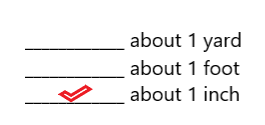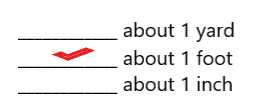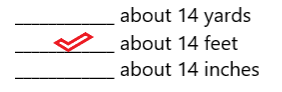# McGraw Hill Math Grade 4 Chapter 10 Lesson 3 Answer Key Customary Units of Length

Practice the questions of McGraw Hill Math Grade 4 Answer Key PDF Chapter 10 Lesson 3 Customary Units of Length to secure good marks & knowledge in the exams.

## McGraw-Hill Math Grade 4 Answer Key Chapter 10 Lesson 3 Customary Units of Length

Solve

Place a check mark next to the best answer.

Question 1.
About how tall is a stamp?Explanation:
The stamp is about 1 inch tall.

Question 2.
About how long is a kite?Explanation:
The kite is about 1 foot tall.

Question 3.
About how long is a car?Explanation:
The car is about 14 feet tall.

Question 4.
About how long is a golf course?Explanation:
The golf course is about 5 miles long.

Multiply to find each missing number.

Question 5.
8 ft = ____________ in.
8 ft = 96 in

Explanation:
1 feet = 12 inches
8 ft = 8 x 12 = 96 inches
So, 8 ft = 96 in.

Question 6.
7 yd = ____________ ft
7 yd = 21 ft

Explanation:
1 yard = 3 feet
7 yards = 7 x 3 = 21 ft
So, 7 yd = 21 ft.

Question 7.
4 mi = ____________ yd
4 mi = 7048 yd

Explanation:
1 mile = 1760 yd
4 miles = 4 x 1760 = 7048 yd
So, 4 miles = 7048 yards.

Question 8.
13 yd = ____________ in.
13 yd = 468 in

Explanation:
1 yard = 36 inches
13 yards = 13 x 36 = 468 in
So,13 yd = 468 in.

Question 9.
75 ft = ____________ in.
75 ft = 900 in

Explanation:
1 feet = 12 inches
75 ft = 75 x 12 = 900 inches
So, 75 ft = 900 in.

Question 10.
6 mi = ____________ ft